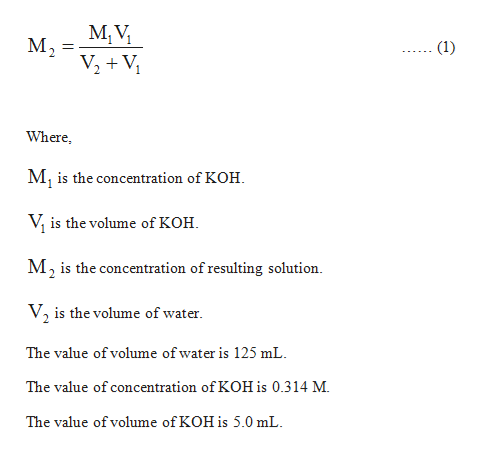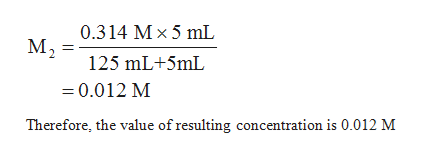# 5.15  If 5.00 mL of 0.314M KOH is diluted to exactly 125 mL with water, what is the concentration of the resulting solution?

Question
1 views

5.15  If 5.00 mL of 0.314M KOH is diluted to exactly 125 mL with water, what is the concentration of the resulting solution?

check_circle

Step 1

The concentration of the substance can be described as the ratio of the moles of the substance to the volume of the solution.

Step 2

The expression to calculate the resulting concentration is shown below:help_outlineImage TranscriptioncloseM, V, M2 V2 + V, (1) Where, M, is the concentration of KOH. V, is the volume of KOH. M, is the concentration of resulting solution. V, is the volume of water. The value of volume of water is 125 mL. The value of concentration of KOH is 0.314 M. The value of volume of KOH is 5.0 mL. fullscreen
Step 3

Substitute the value in ...help_outlineImage Transcriptionclose0.314 Mx 5 mL м, 125 mL+5mL = 0.012 M Therefore, the value of resulting concentration is 0.012 M fullscreen

### Want to see the full answer?

See Solution

#### Want to see this answer and more?

Solutions are written by subject experts who are available 24/7. Questions are typically answered within 1 hour.*

See Solution
*Response times may vary by subject and question.
Tagged in

### Chemistry